## 从HashMap说起

HashMap是上面提到的几个Map中使用频率最高的了，毕竟需要考虑到多线程并发的场景并不算太多。下面是Map的一个关系图，大家了解一下即可。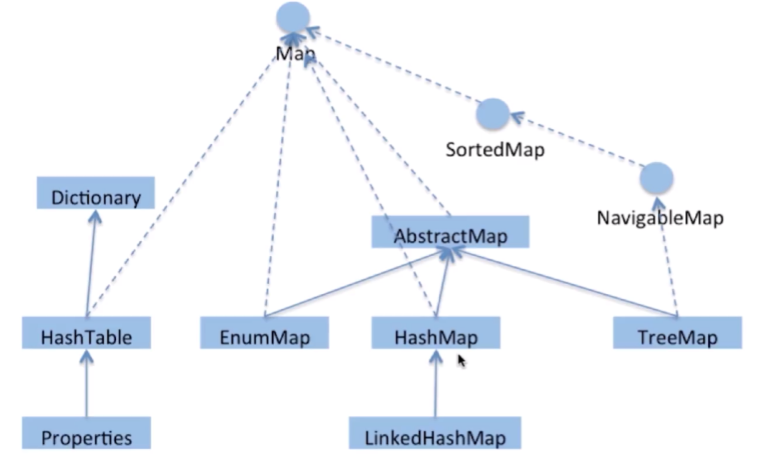HashMap在Java8之前和之后有很大差别，在Java8以前，它的数据结构是数组+链表的形式，8以后就变成了数组+链表+红黑树的结构。它的key是保存在一个Set里面的，也就是有去重的功能，values是存在一个Collections里面。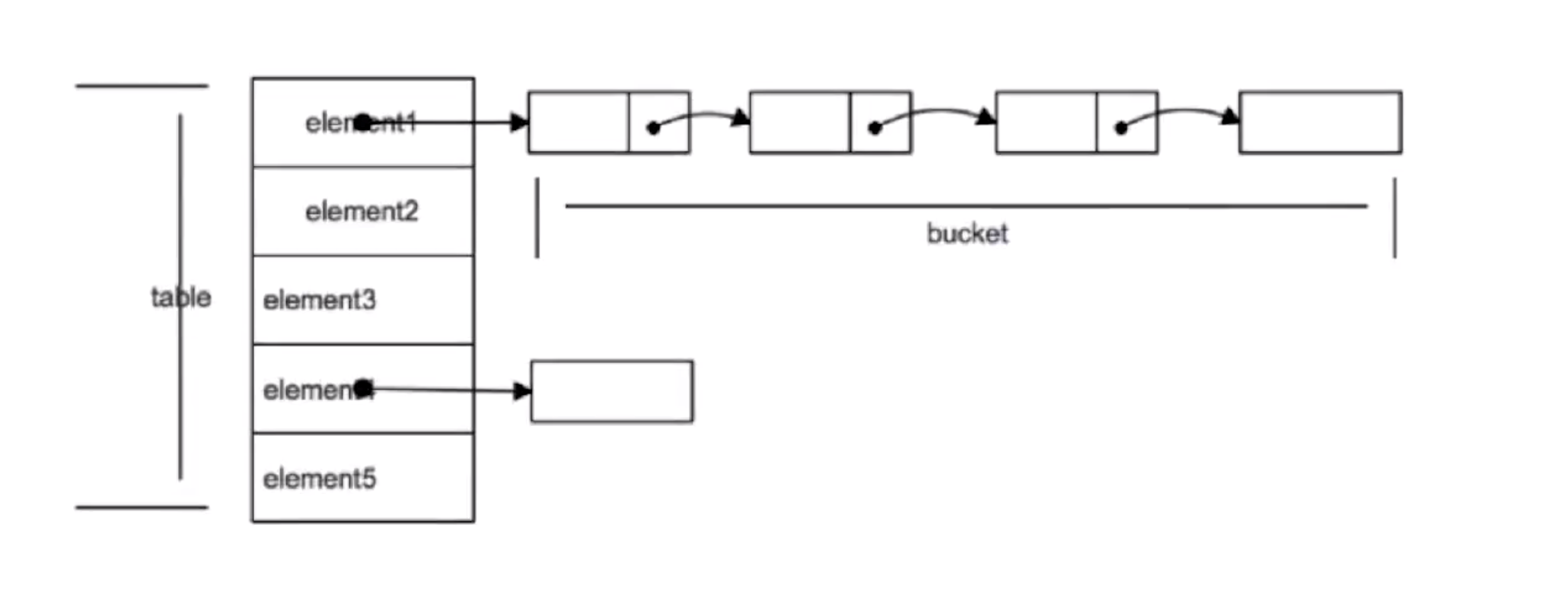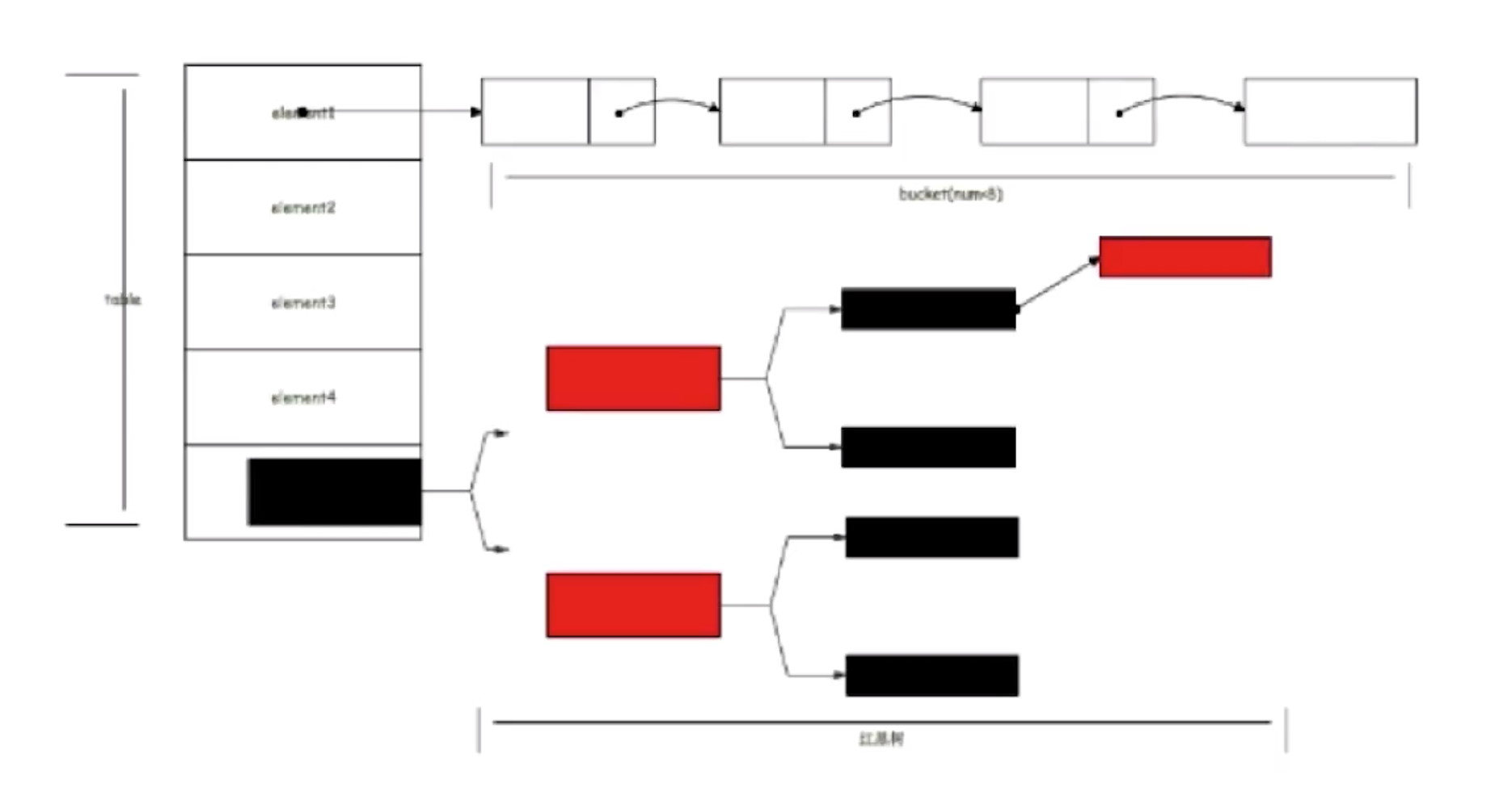HashMap里的数组每个元素存放的是key-value形式的实例，Java7里面叫做Entry，8里面叫Node。这个Node里面包含了hash值，键值对，下一个节点next这几个属性组成。数组被分为一个个bucket，也就是桶，通过hash值决定了键值对在这个数组中的寻址，hash值相同的则以链表的形式存储，链表长度超过阈值就转成红黑树。那么先来看看HashMap的put操作，

final V putVal(int hash, K key, V value, boolean onlyIfAbsent,
boolean evict) {
Node<K,V>[] tab; Node<K,V> p; int n, i;
//为空则初始化
if ((tab = table) == null || (n = tab.length) == 0)
n = (tab = resize()).length;
// 算出键值对在table中的具体位置，没有就new一个node
if ((p = tab[i = (n - 1) & hash]) == null)
tab[i] = newNode(hash, key, value, null);
else {
// 如果存在
Node<K,V> e; K k;
//一样就替换
if (p.hash == hash &&
((k = p.key) == key || (key != null && key.equals(k))))
e = p;
//树化了就用树的形式保存
else if (p instanceof TreeNode)
e = ((TreeNode<K,V>)p).putTreeVal(this, tab, hash, key, value);
else {
//链表的形式插入元素
for (int binCount = 0; ; ++binCount) {
if ((e = p.next) == null) {
p.next = newNode(hash, key, value, null);
if (binCount >= TREEIFY_THRESHOLD - 1) // -1 for 1st
treeifyBin(tab, hash);
break;
}
if (e.hash == hash &&
((k = e.key) == key || (key != null && key.equals(k))))
break;
p = e;
}
}
// 存在就更新
if (e != null) { // existing mapping for key
V oldValue = e.value;
if (!onlyIfAbsent || oldValue == null)
e.value = value;
afterNodeAccess(e);
return oldValue;
}
}
++modCount;
//超过阈值就扩容
if (++size > threshold)
resize();
afterNodeInsertion(evict);
return null;
}


1. 若HashMap未被初始化，则进行初始化操作
2. 对Key求Hash值，依据Hash值计算下标
3. 若未发生碰撞，则直接放入桶中
4. 若发生碰撞，则以链表的方式链接到后面
5. 若链表长度超过阈值，且HashMap元素超过最低树化容量，则将链表转成红黑树
6. 若节点已经存在，则用新值替换旧值
7. 若桶满了，就需要resize（扩容2倍后重排）

HashMap的初始容量是多少？为什么设置成这个值呢？

/**
* The default initial capacity - MUST be a power of two.
*/
static final int DEFAULT_INITIAL_CAPACITY = 1 << 4; // aka 16


/**
* The load factor used when none specified in constructor.
*/
static final float DEFAULT_LOAD_FACTOR = 0.75f;


put的时候会先判断是否碰撞，那么如何减少碰撞呢？

### SynchronizedMap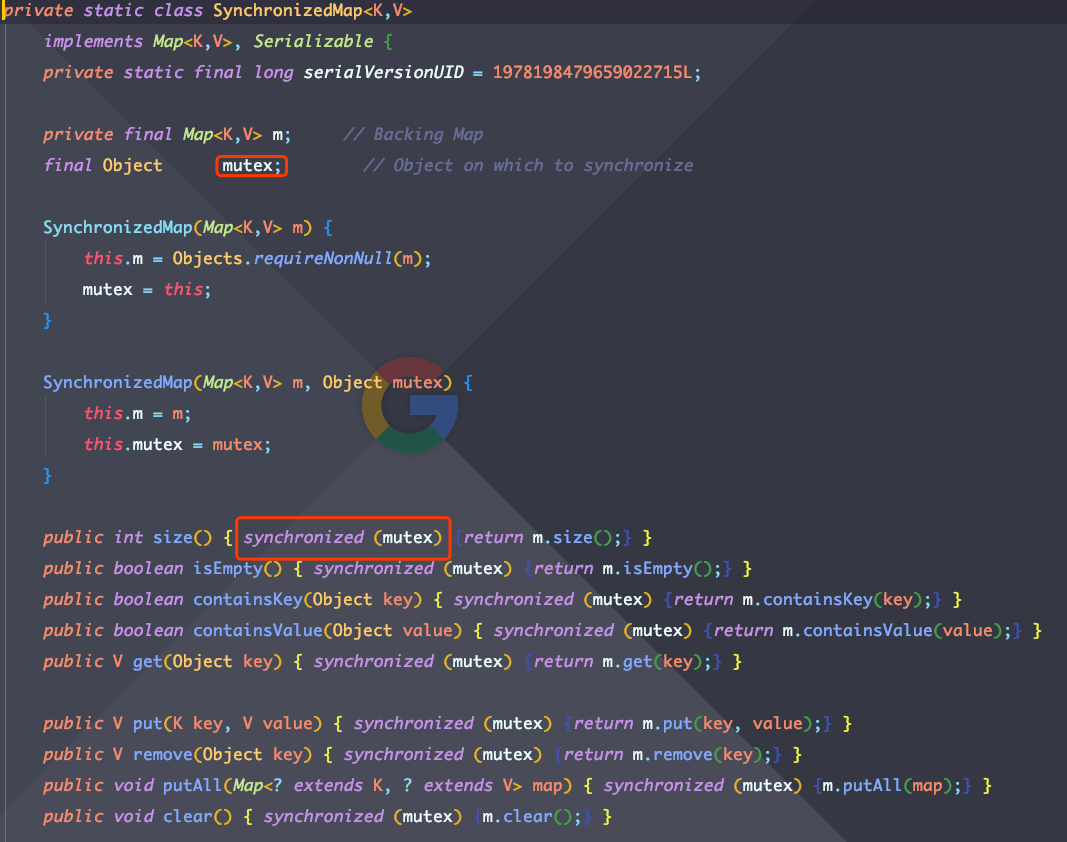## ConcurrentHashMap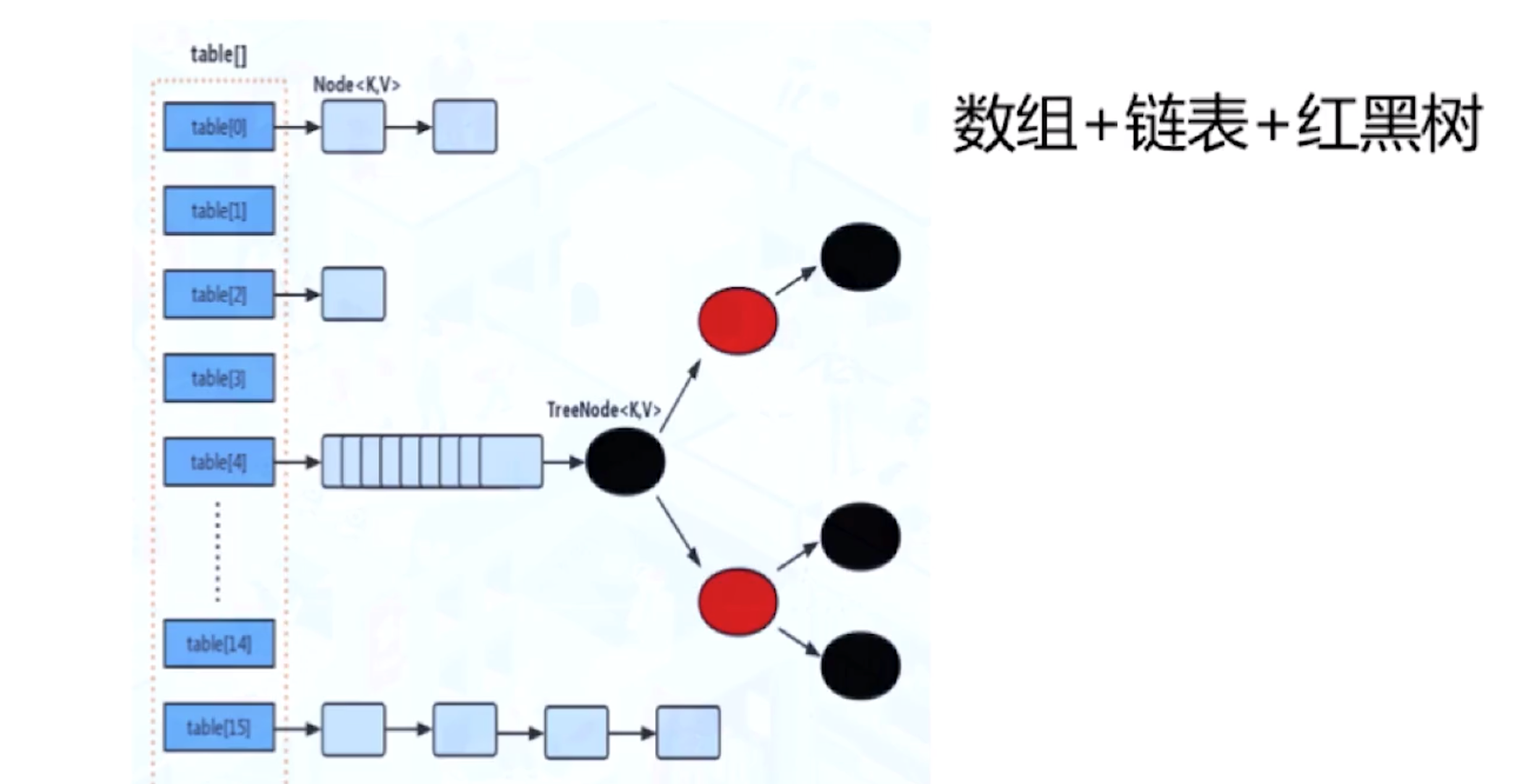synchronized锁住当前链表或者红黑树的首节点，这样只要哈希不冲突，就不会出现并发问题。

/**
* Table initialization and resizing control.  When negative, the
* table is being initialized or resized: -1 for initialization,
* else -(1 + the number of active resizing threads).  Otherwise,
* when table is null, holds the initial table size to use upon
* creation, or 0 for default. After initialization, holds the
* next element count value upon which to resize the table.
*/
private transient volatile int sizeCtl;


ConcurrentHashMap和HashMap的参数差不多，但有些特有的，比如sizeCtl。它是哈希表初始化或扩容时的一个控制位标识量，负数代表正在初始化或正在扩容操作。同样的，我们也看看它的put操作。

final V putVal(K key, V value, boolean onlyIfAbsent) {
// 键值都不能为null
if (key == null || value == null) throw new NullPointerException();
//计算key的hash值
int binCount = 0;
// 数组元素的更新，使用CAS，所以需要不断失败重试
for (Node<K,V>[] tab = table;;) {
Node<K,V> f; int n, i, fh;
if (tab == null || (n = tab.length) == 0)
// 初始化
tab = initTable();
//找到f，即链表或者红黑树的头节点，没有就添加
else if ((f = tabAt(tab, i = (n - 1) & hash)) == null) {
//CAS添加，失败break
if (casTabAt(tab, i, null,
new Node<K,V>(hash, key, value, null)))
break;                   // no lock when adding to empty bin
}
// 如果正在移动元素，就协助扩容
else if ((fh = f.hash) == MOVED)
tab = helpTransfer(tab, f);
else {
//发生hash碰撞，锁定链表或者红黑树的头节点f
V oldVal = null;
synchronized (f) {
// 判断f是否时链表的头节点
// fh就是头节点的hash值
if (tabAt(tab, i) == f) {
if (fh >= 0) {
//如果是，初始化链表的计数器
binCount = 1;
for (Node<K,V> e = f;; ++binCount) {
K ek;
//节点存在就更新
if (e.hash == hash &&
((ek = e.key) == key ||
(ek != null && key.equals(ek)))) {
oldVal = e.val;
if (!onlyIfAbsent)
e.val = value;
break;
}
// 不更新就插入
Node<K,V> pred = e;
if ((e = e.next) == null) {
pred.next = new Node<K,V>(hash, key,
value, null);
break;
}
}
}
//头节点是红黑树的头，用红黑树的方式插入
else if (f instanceof TreeBin) {
Node<K,V> p;
binCount = 2;
if ((p = ((TreeBin<K,V>)f).putTreeVal(hash, key,
value)) != null) {
oldVal = p.val;
if (!onlyIfAbsent)
p.val = value;
}
}
}
}
if (binCount != 0) {
//链表长度达到了8，则转换成树结构
if (binCount >= TREEIFY_THRESHOLD)
treeifyBin(tab, i);
if (oldVal != null)
return oldVal;
break;
}
}
}
// ConcurrentHashMap的size+1
return null;
}


1. 判断Node[]数组是否初始化，没有则进行初始化操作
2. 通过hash定位数组的索引坐标，是否有Node节点，如果没有则使用CAS进行添加（链表的头节点），添加失败则进入下次循环
3. 检查到内部正在扩容，就帮助它一块扩容
4. 如果头节点f！=null,则使用synchronized锁住f元素（链表/红黑二叉树的头元素）
• 如果是Node（链表结构）则进行链表的添加操作
• 如果是TreeNode结构则执行树添加操作
5. 判断链表长度已经达到临界值8，这个8可以自己调整，当节点数超过这个值就把链表转换为树结构

## HashMap、HashTable以及ConcurrentHashMap的区别

• HashMap线程不安全，数组+链表+红黑树
• HashTable线程安全，锁住整个对象，数组+链表
• ConcurrentHashMap线程安全，CAS+同步锁，数组+链表+红黑树
• HashMap的key，value均可为null，其他两个不可以
• HashTable使用的是安全失败机制（fail-safe），这种机制会使你此次读到的数据不一定是最新的数据。如果你使用null值，就会使得其无法判断对应的key是不存在还是为空，因为你无法再调用一次contain(key）来对key是否存在进行判断，ConcurrentHashMap同理
• HashMap 的初始容量为：16，Hashtable 初始容量为：11，两者的负载因子默认都是：0.75。
• 当现有容量大于总容量 * 负载因子时，HashMap 扩容规则为当前容量翻倍，Hashtable 扩容规则为当前容量翻倍 + 1。
• HashMap 中的 Iterator 迭代器是 fail-fast 的，而 Hashtable 的 Enumerator 不是 fail-fast 的。
• 快速失败（fail—fast）是java集合中的一种机制， 在用迭代器遍历一个集合对象时，如果遍历过程中对集合对象的内容进行了修改（增加、删除、修改），则会抛出Concurrent Modification Exception。

## 发送评论编辑评论

|´・ω・)ノ
ヾ(≧∇≦*)ゝ
(☆ω☆)
（╯‵□′）╯︵┴─┴
￣﹃￣
(/ω＼)
∠( ᐛ 」∠)＿
(๑•̀ㅁ•́ฅ)
→_→
୧(๑•̀⌄•́๑)૭
٩(ˊᗜˋ*)و
(ノ°ο°)ノ
(´இ皿இ｀)
⌇●﹏●⌇
(ฅ´ω`ฅ)
(╯°A°)╯︵○○○
φ(￣∇￣o)
ヾ(´･ ･｀｡)ノ"
( ง ᵒ̌皿ᵒ̌)ง⁼³₌₃
(ó﹏ò｡)
Σ(っ °Д °;)っ
( ,,´･ω･)ﾉ"(´っω･｀｡)
╮(╯▽╰)╭
o(*////▽////*)q
＞﹏＜
( ๑´•ω•) "(ㆆᴗㆆ)

Emoji